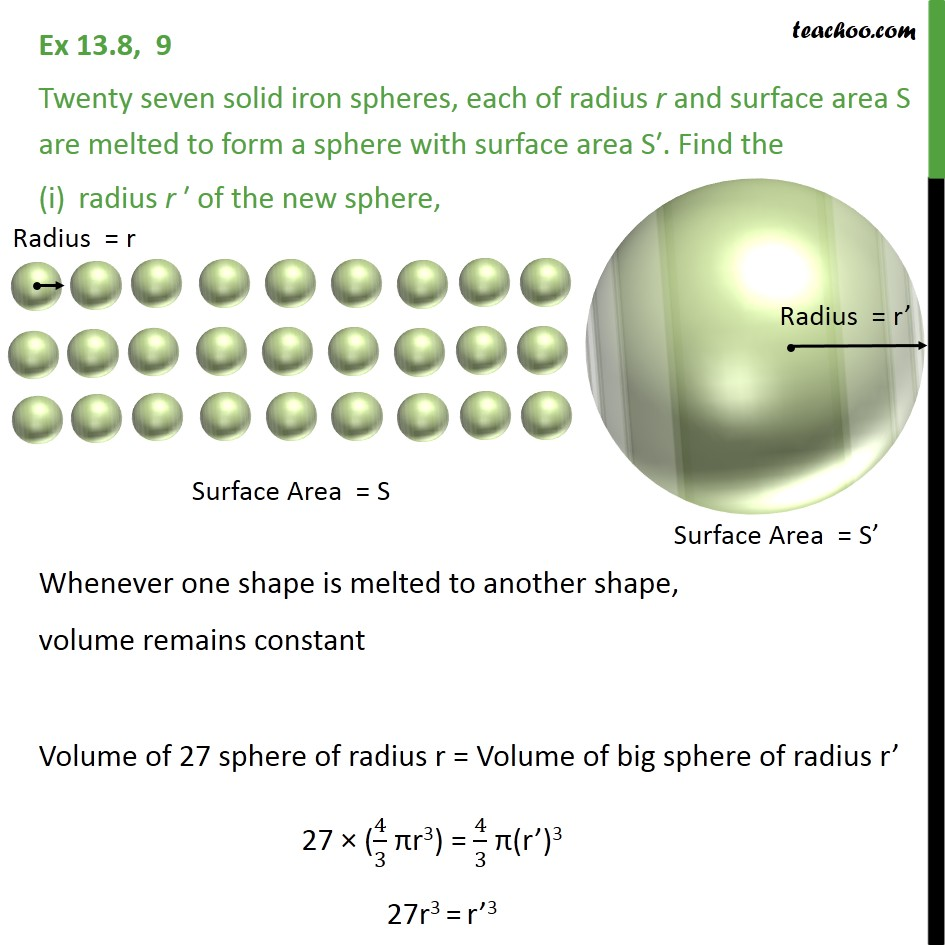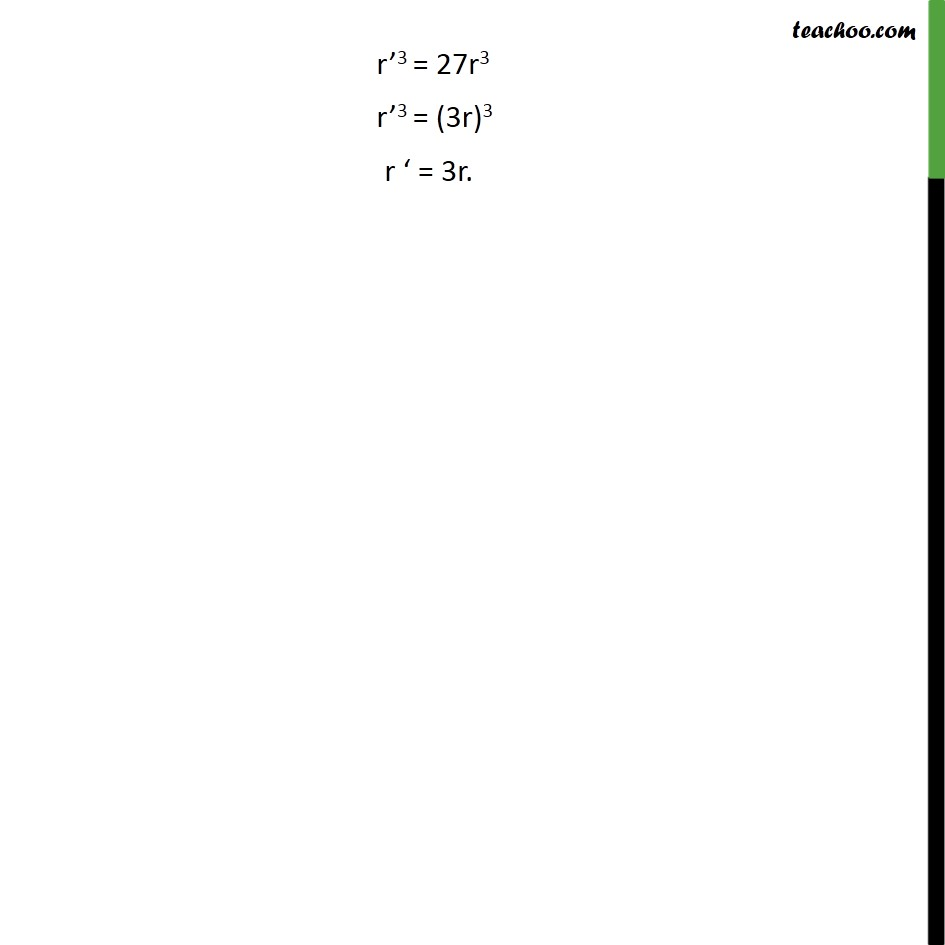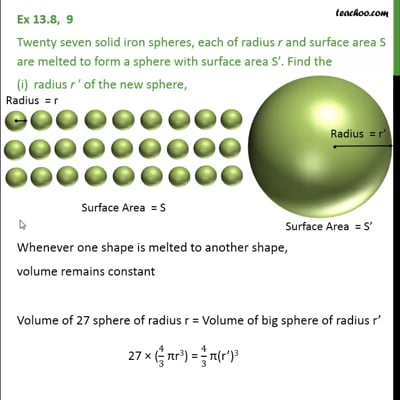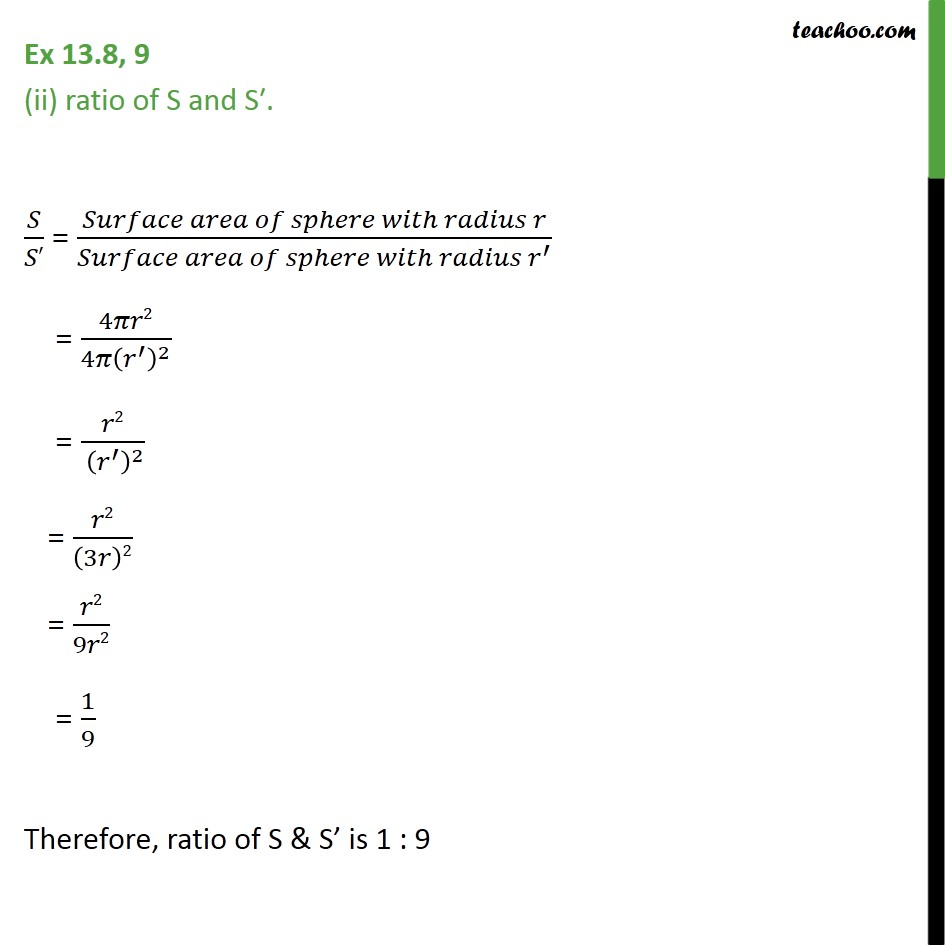Ex 13.8

Chapter 13 Class 9 Surface Areas and Volumes
Serial order wiseThis video is only available for Teachoo black usersThis video is only available for Teachoo black users

Maths Crash Course - Live lectures + all videos + Real time Doubt solving!

### Transcript

Ex 13.8, 9 Twenty seven solid iron spheres, each of radius r and surface area S are melted to form a sphere with surface area S . Find the radius r of the new sphere, Whenever one shape is melted to another shape, volume remains constant Volume of 27 sphere of radius r = Volume of big sphere of radius r 27 (4/3 r3) = 4/3 (r )3 27r3 = r 3 r 3 = 27r3 r 3 = (3r)3 r = 3r. Ex 13.8, 9 (ii) ratio of S and S . / = ( )/( ^ ) = 4 2/(4 ( ^ )^2 ) = 2/( ( ^ )^2 ) = 2/(3 )2 = 2/9 2 = 1/9 Therefore, ratio of S & S is 1 : 9